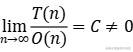「数据结构」算法效率度量--时间（空间）复杂度详解

目录

一、算法的基本概念

2、算法的五个特性

• 有穷性。一个算法必须总在执行有穷步之后结束，且每一步都可在有穷时间内完成。

• 确定性。算法中每条指令必须有确切的含义，对于相同的输入只能得到相同的输出。

• 有穷性。算法中描述的操作都可以通过已经实现的基本运算执行有限次来实现。

• 有穷性。一个算法有零个或多个输入，这些输入取自于某个特定的对象的集合。

• 有穷性。一个算法有一个或多个输出，这些输出是与输入有着某种特定关系的量。、

3、“好算法”的4个目标

• 正确性。算法应能正确的解决问题。

• 可读性。算法应具有良好的可读性，以帮助人们理解。

• 健壮性。输入非法数据时，算法能适当地作出反应或进行处理，而不会产生莫名其妙的输出结果。

• 效率与低存储需求。效率是指算法执行的时间，存储量需求是指算法执行过程中所需要的最大存储空间，这两者都与问题的规模有关。

二、算法效率的度量

1、时间复杂度

T(n) ：算法中所有的语句频度之和记作T（n）,它是该算法问题规模n的函数，时间复杂度主要分析T（n）的数量级。

O(n)：语句频度总和T(n)的数量级。用数学表达式即T(n)与O(n)是同阶无穷大2、分析程序的时间复杂性遵循以下两条规则

• 加法规则

T(n) = T1(n) + T2(n) = O(f(n)) + O(g(n)) = O(max(f(n),g(n)));

• 乘法规则

• T(n) = T1(n)T2(n) = O(f(n))×O(g(n)) = O(f(n)×g(n))

3、常见的渐进时间复杂度

O(1) ＜ O(㏒2(n)) ＜ O(n) ＜ O(n㏒2(n)) ＜ O(n²) ＜ O(n³) ＜ O(2ⁿ) ＜ O(n!) ＜ O(nⁿ)

S(n)=O(g(n))

三、时间复杂度的分析实例

x=90;y=100;
while(y>0){
if(x>100){
x=x-10;
y--;
}
else {
x++;
}
}

2、乘法规则O(mn)

for(int i=0;i<n;i++){
for(int j=0;j<m;j++){
a[i][j]=0;
}
}

3、平方阶O(n²)

s=0;
for(int i=0;i<n;i++){
for(int j=0;j<n;j++){
s++;
}
}

4、对数阶O(㏒3(n))

• 做法

设while语句循环t次，则：

i=1、3、9、27、81……

t=0、1、2、3、4……

立即推：i=3^t ==>(while 循环i<=n得出) 3^t<n 解出t=㏒3(n)

所以有：T(n)=O(t)=O(㏒3(n))

i=1;
while(i<=n){
i=i*3;
}